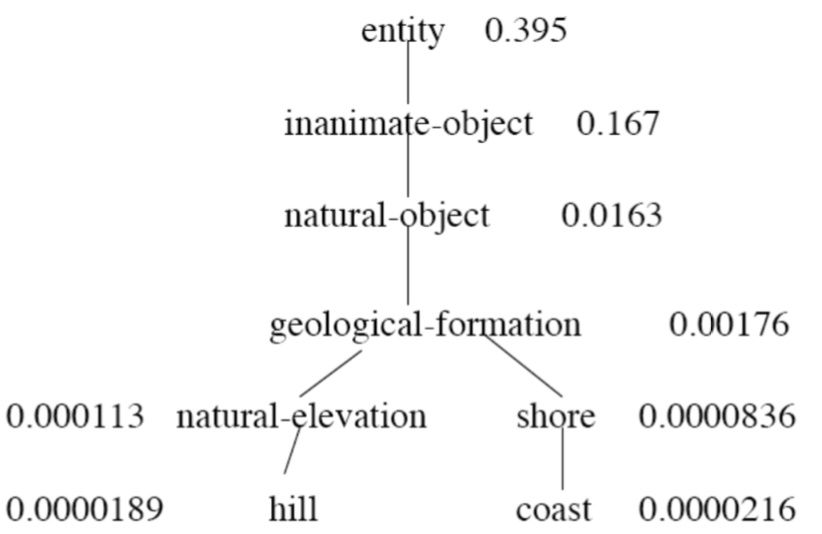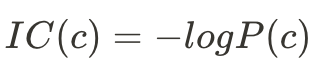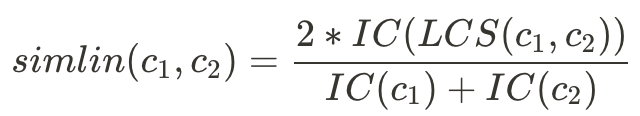#DAY 28
3
AI & Data

## Day 28: 文字相似度- 語言學

"This is a good movie." → :)
"This is a great movie." → :)
"This is a bad film." → :(
"This is a wonderful film." → ?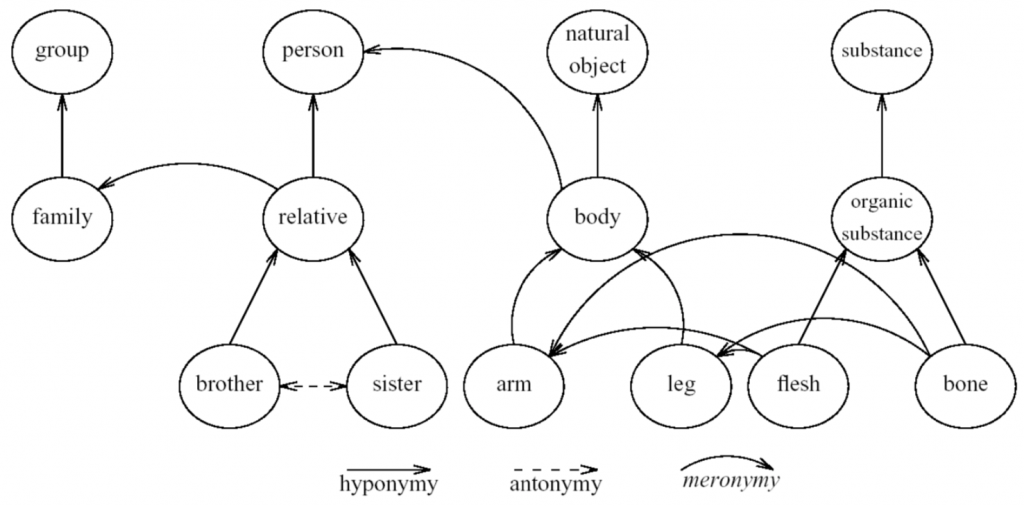• hyponym: 上位詞 < - > hypernym: 下位詞
• antonym: 反義詞 < - > synonym: 相似詞
• meronym: 局部詞 < - > holonym: 整體詞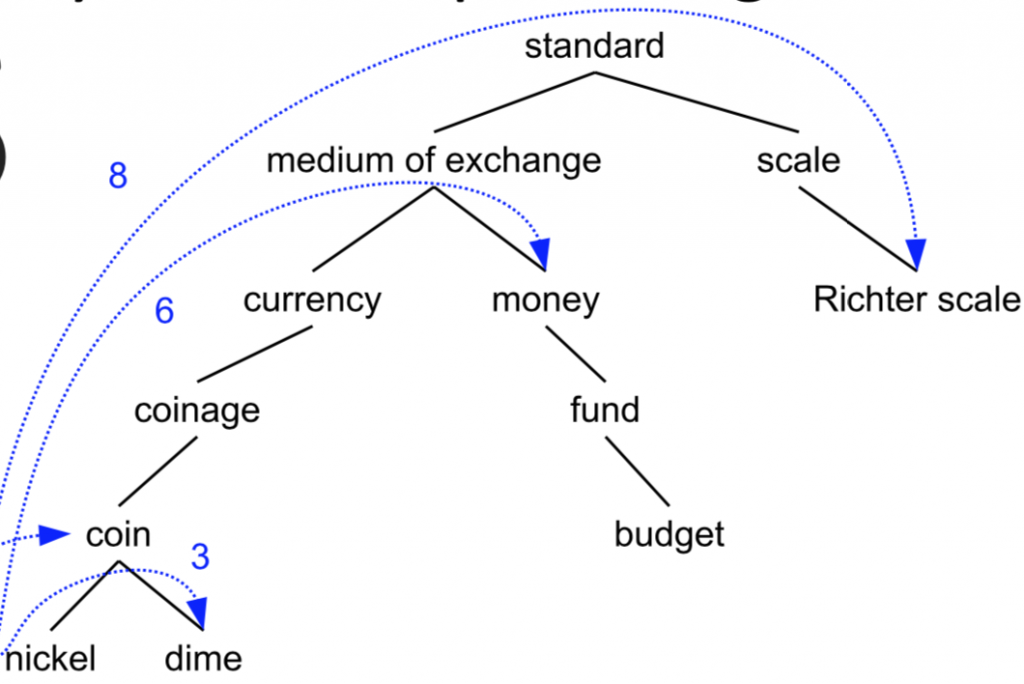1. 根據關係距離取得相似度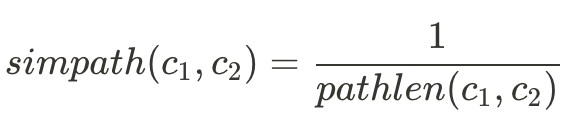• simpath(nickel, nickel) = 1 / 1 = 1
• simpath(nickel, coin) = 1 / 2 = 0.5
• simpath(nickel, money) = 1 / 6 = 0.17
• simpath(nickel, Richter scale) = 1 / 8 = 0.125
1. Wu & Palmer相似度，把語義深度（在資料庫中的深度）也列入考量：• simwup(nickel, money) = 2*2 / (3+6) = 0.44
• simwup(nickel, Richter scale) = 2*1 / (3+6) = 0.22
1. Lin Similarity，將文字出現在文集中的機率也考慮進去：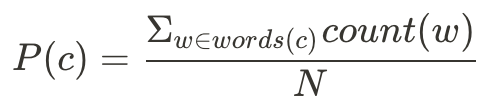P(c)將所有包含這個字的文字（文字關係在c字之下的所有字，如P(coin)中的w={coin, nickel, dime}），N則為所有文集中的字數。